### Pressure Sensors

Differential Pressure Sensors

Absolute Pressure Sensors

Barometric Pressure Sensors

Compound Pressure Sensors

Gauge Pressure Sensors

Submersible Pressure Sensors

Vacuum Pressure Sensors

UHP Pressure Sensors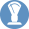### Environmental Monitoring System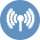### Wireless Sensors### Room Pressure Monitors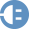### Power Meters & Accessories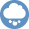### Humidity/Temp Sensors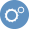### Pressure Calibrator### Particle Counters### Dolan-Jenner Fiber Optics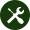### Building Automation

Calibration

HVAC/R OEM

HVAC/R### Energy Management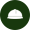### Critical Environments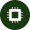### Semiconductor Manufacturing### Data Centers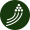### Fiber Optics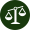### Scales### General Industrial

Alternative Fuels

Barometric

Data Centers

General Industrial OEM

HVAC/R OEM

Medical

Off-Highway Vehicles

Oil & Gas

Process / MFG Tank Level

Test & Measurement

Water & Wastewater

March 30, 2015

#### by Brandon Rogers

Alternating Current - current that reverses its direction of flow periodically (Hz).

Ampere - a unit of measure for the rate of current flow

##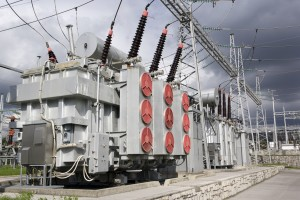Apparent Power - applied voltage multiplied by current in an AC circuit. This value would not take the power factor into account. Unit is volt amperes. (VA).

Balanced Load - AC power system using more than two wires, where the current and voltage are of equal value in each energized conductor.

Bandwidth - the range of frequencies over which an instrument provides accurate measurement

Billing Consumption - total amount of energy consumed during a predetermined period(usually 28-33 days).

Consumption (active energy) - actual electrical energy used measured in kilowatt hours (kWh) by the watt hour meter, regardless of the power factor.

Crest Factor - the ratio of the peak value of a wave form (voltage or current) to the RMS value.

Current Transformer - an instrument accessory which detects current flow without breaking
the circuit under test. An AC transformer, usually
step-down; typical ratio listing would be 1000:1. This would indicate 1000A on the primary and 1A on the secondary.

Current Transformer Ratio - the ratio of primary amperes divided by secondary amperes.

Delta Connection - a circuit formed by connecting three electrical devices in series to form
a closed loop; most often used in three-phase connections.

Demand (active, real or true power) - the power which is actually consumed by the load. The measurement takes the power factor into account.

Derating Factor - a number defined as 1.414 x average RMS phase current/peak phase current. This factor, when applied to the rated load of a transformer, gives an indication as to the percent loading that is reasonable when that transformer must service nonlinear loads.

Displacement Power Factor - the difference between apparent power and true power when only the phase relationship of voltage and current at the fundamental are taken into account.

Distortion Factor (%DF) - total harmonic Distortion referenced the total RMS signal (THD-R).

Distortion Power Factor - the difference between apparent power and true power frequencies.

Frequency - the number of complete cycles of AC voltage which occurs during one second (Hz).

Harmonics - current or voltages which have frequencies that are integer multiples of the fundamental power frequency; common and sometimes dangerous in nonlinear loads.

Heating Effect - temperature increase in electrical distribution equipment caused by an increase in RMS current.

Impedance - the total opposition to alternating current flow in an electrical circuit (Z).

Inductive Reactance - the force which acts as a resistance in an inductor to limit the flow of current. The force creates a leading power factor in AC circuits.

Initiator Pulses - electrical impulses generated from utility revenue meters. Each pulse indicates a specific number of watts consumed. These pulses are used within energy analyzers to measure energy consumption and demand.

K Factor - a number based on the harmonic content of load current that determines the maximum safe loading on a power source.

K-Rated Transformers - a transformer that is rated or designed to serve as the source for a predefined capacity of harmonic current.

Peak Demand (maximum RMS power) - the highest average load during a specified time interval (kW).

Phase - time relationship between current and voltage in AC circuits.

Potential Transformer - an instrument transformer used to step down high voltage potentials to lower levels acceptable for the input of electrical test instruments.

Power Factor - the ratio of true power (watts) to apparent power (volt amperes). Expressed in decimal form, e.g. .98.

Ratchet Demand - determining the billing demand based upon a pre-established peak average
demand (usually at 75%, 80% or 100% of the pre-established peak).

Reactance - the opposition to current flow in an AC circuit introduced through inductance or capacitance.

Reactive Compensation Power - the reactive power to be applied to an AC network for power factor correction; adding capacitance in order to bring the voltage and current waveform in phase.

Reactive Power (kvar) - power which is actually “borrowed” from the load and returned to the power source each cycle; unused power.

Resolution - the smallest unit value that an instrument can measure.

Resonance - when the inductance in the system and the natural capacitance of the system, or added capacitors, form a tuned circuit resonant at one or more of the harmonic frequencies produced by nonlinear loads.

RS-232 - a computer interface connector used to connect serial devices such as instruments for information transfer.

Sensitivity - the smallest input that will provide a specified output.

Skin Effect - phenomenon in which high harmonic frequencies cause electrons to flow to the outer sides of a conductor, reducing its cross-sectional diameter, and hence its ampacity rating.

Sliding Demand - calculating average demand by averaging the average demand over several
successive time intervals, advancing one interval at a time.

THD (%THD, Total Harmonic Distortion) - the contribution of all harmonic frequency currents or voltages to the fundamental current or voltage, expressed as a percentage of the fundamental.

THDF (Transformer Harmonic Derating Factor) - method of calculating transformer derating established by CBEMA for phase-to-neutral loads.

True RMS - capability to accurately measure the value of AC voltage and current having a non sinusoidal waveform as well as sinusoidal wave forms.

Unbalanced Load - an AC power system using more than two wires where the current is not equal in the current-carrying wires due to an uneven loading of the phases.

Watt - the measure of real power. It is the power expended when one ampere of direct current flows through a resistance of one ohm.

Wye Connection - a connection of three components made in such a manner that one end of each component is connected; generally used to connect devices to a three-phase power system.